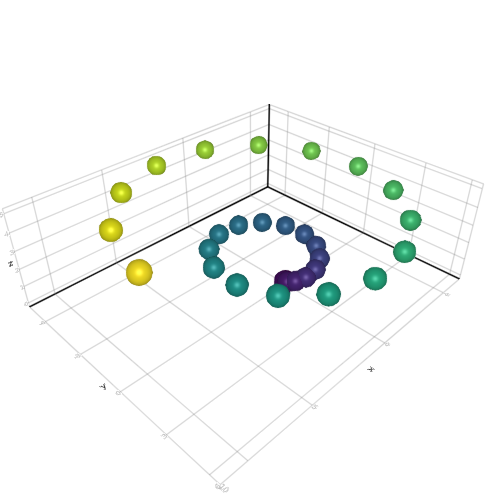## Viridis meshscatter

```

using AbstractPlotting

N = 30
R = 2
theta = 4pi
h = 5
x = [R .* (t/3) .* cos(t) for t = range(0, stop = theta, length = N)]
y = [R .* (t/3) .* sin(t) for t = range(0, stop = theta, length = N)]
z = range(0, stop = h, length = N)
meshscatter(x, y, z, markersize = 0.5, color = to_colormap(:viridis, N))

```